﻿ The Determinants of Bank Performance: The Case of Tunisian Listed BanksPublications are Open
Access in this journal
Article Versions
Export Article
• Normal Style
• MLA Style
• APA Style
• Chicago Style
Research Article
Open Access Peer-reviewed

The Determinants of Bank Performance: The Case of Tunisian Listed Banks

Aziz Chouikh , Youssef Blagui
Journal of Finance and Accounting. 2017, 5(2), 53-60. DOI: 10.12691/jfa-5-2-4
Published online: July 05, 2017

Abstract

This paper studies the relationship between Tunisian listed banks performance and two types of determinants; internal and external. The internal explanatory variables are: (1) the bank size, (2) privatization, (3) board size, (4) capital-to-assets ratio, and (5) cost of efficiency. The macro-economic (external) exogenous variables are: (1) gross domestic product growth rate and (2) inflation. Our panel-data analyses suggest a statistically significant and negative relationship between bank profitability (endogenous variable) and board size. However, the remaining variables were found to be statistically insignificant. This can be explained by two main sub-hypotheses: (a) state-owned banks included in the sample disturb the statistical significance of the results and (b) the year 2011 is a cut-off point that changed the Tunisian bank performance determinants.

1. Introduction

Bank performance is a key lever for any country financial development. In a continuously changing environment{1}, understanding its determinants would act as an insurance against any risk of economic deterioration. Whether they are state-owned or private, these financial institutions play an important role in safeguarding individual’s money and providing loans for investors. However, if they do not perform well, these would lead to a critical financial crisis such as the sub-prime mortgage crisis in 2008. Not only would that harm the global environment, but consequences would also last for years. In this context, having a clear idea about what causes the bank profitability would allow Tunisian banks to boost their country’s economy, especially, after the revolution of 2011. In the past years, Tunisia has suffered from instable financial conditions that have pushed away investors. Consequently, bank performance has been impacted and investment projects have been blocked. This paper aims to empirically investigate the determinants of Listed Tunisian banks performance. For this purpose, we will study the impact of two types of variables: internal and macro-economic. The internal explanatory variables are: (1) the bank size, (2) privatization, (3) board size, (4) capital-to-assets ratio, and (5) cost of efficiency. The macroeconomic exogenous variables are (1) gross domestic product (GDP) growth rate and (2) inflation.

The remainder of this paper is organized as follows: section 2 presents the art of the empirical literature. Section 3 points out the variables. Section 4 sets up the empirical methodology. Section 5 deals with the model estimations. Section 6 copes with the results and findings. Finally, in section 7, we conclude.

2. Literature Review

The bank capital theory suggests that banks are able to survive with higher capital and less liquidity (Diamond and Rajan 24). Several empirical studies have been made about this positive relationship. In addition, empirical evidence has also been reported about the positive relationship between the return on equity and capital-to-asset ratio. Another study made by Abreu and Mendes 1 showed that European banks with higher capital performed better than competitors with lower one. Not only could they face bankruptcy risk, but also they could rely much less on external funding sources. Similar studies supported the same findings such as Abreu & Mendes 1, Liu et al 43, Bourke 17, and Goddard et al 30. However, researchers Hughes and Mester 33 found antagonist results. In fact, they found that higher capital-to-assets ratio results in increased variable costs, which do not really translate in higher profitability. Furthermore, Clementina and Isu 21 consistently reported that improved capital position enhances the bank performance.

Economic literature, theoretical as well as empirical, has connected the performance of banks to competition, concentration, efficiency, productivity, and profitability (See Jaap and Bikker 34). The economic theory indicates an inverted relationship between bank profitability and efficiency cost. However, empirical researches suggest antagonist results. An empirical study made by Košak and Zajc 40 proved that there is a positive relationship between the two variables when it comes to banks belonging to European Union. Similar researches made by Sanchez et al 56, Kasman & Carvallo 38, and Berger and Mester 15 pointed out the same findings. Banks with better cost of efficiency tend to optimize the allocation of their resources, which results in higher income.

The Productive Efficiency theory suggests that private-ownership lead to the implementation more efficient policies. The effect of this variable has been tackled by several researchers such as Makhoha 44 and Beck et al 6. The latter studied the effect of a private ownership over Nigerian Banks. For this objective, they collected annual data of more than 68 banks. They decided to cover a period of 11 years which led to more than 500 observations. Results showed that privatized banks were more performing. Similar studies performed by Dietrich and Wanzenried 25 over Swiss banks support the same findings. However, they empirically proved the negative impact of private-ownership on banks performance following the study of more than 115 countries.

Investigators such as Ben Naceur and Ghazouani 12 and Umar et al 66 were in favor of an inverse link between the inflation and bank performance. The more inflation increases, the less the banking sector shows positive results and vice versa. This conclusion has been made after a panel data study over 11 countries from the MENA{3} regions. Similarly, Boyd et al 18 evaluated the effect on inflation of banking belonging to more than 95 countries. To do so, they collected data that cover a period of 35 years. Their panel data estimation led to the statistical evidence of a negatively significant relationship. Nevertheless, Clementina et al 22 found out a positive but statistically insignificant relationship between inflation and bank performance. Other studies made by Naceur and Kandil 47 evidenced the same empirical results. However, this outcome was empirically rejected by authors such as Molyneux and Thornton 45, Pasiouras and Kosmidou 50, and Revell 53. In addition, researchers such as Asutay and Izhar 4 collected data about a sample of Indonesian Islamic banks and regressed the dependent variable, which was return on assets (ROA), on the independent variable inflation. As a result, they found out that inflation has a positive impact on these bank profitability measures.

Economic theory suggests a positive relationship between economic growth and bank profitability (Calza et al, 20). Murad et al 46 empirically founded out a statistically significant relationship between bank performance and interna and external determinants. Besides, it was associated positively with bank performance by Beckmann 7, Goddard et al  31, and Arpa et al 3. They believe that an increase GDP would increase the consumer purchasing power. As a consequence, people would tend to get more credits and invest on a higher frequency. That would result in an increase of cash inflow leading to better bank performance.

3. The Variables

3.1. The Endogenous Variables

We deal with three dependent variables that are used as proxies of the bank performance. corresponding author. corresponding author.

3.1.1. Return on Assets (ROA)

Most of literatures refer to the return on asset (ROA) as a measure of bank profitability. Researchers such as Nippani and Green 48 that validated its usefulness in having an approximation of bank profitability. Furthermore, it has been used by Beck et al 6 to estimate the profitability of Nigerian banks as well as Asutay and Izhar 4 to evaluate Indonesian bank performance. It is measured by the ratio Net income to Total assets. It shows the profit earned per 1 dinar of assets. In other words, it is an indicator of how well a bank uses it assets in order to generate profit. ROA can be directly derived from bank’s financial statements or calculated using the ratio stated above.

3.1.2. Return on Equity (ROE)

Return on equity is considered by most of the literatures in order to calculate the bank performance. For instance, It has been used by Berger and Humphrey 14, Ganesan 29, Fries and Taci 28, and De Young and Rice 23. It is measured by the ratio of net income to stockholder equity. This ratio shows the profit earned per 1 dinar of investment. It is an indicator of how well bank uses investors’ money to generate profit. ROE can be directly derived from bank’s financial statements or calculated using the ratio stated above.

3.1.3. Price to Book Ratio (PB)

It is equal to the ratio of market stock close price to book value per share. It is done on an annual basis meaning that the close price is taken on December 31st of each year. A ratio lower than 1 indicates a value destruction; however, a ratio greater than 1 means a value creation.

These explained variables are summarized in the table below.

3.2. The Exogenous Variables

They can be split into two categories: internal and macroeconomic (i.e. external) variables. We cope with five internal explanatory variables, while the macroeconomic variables are two.

3.2.1. Internal Exogenous Variables

Five exogenous variables specific to the banks are chosen. The first one is the board of size (Bos) which is measured by the number of directors that are officially board members. Then, the bank size (Bs), which is measured by the logarithm of total assets, will be used. Furthermore, we will refer to the cost of efficiency (Ceff) which is equal to the ratio of overhead cost to bank total assets. In addition, private-ownership (Priv) will be considered. It is a dummy variable that is equal to 1 when the bank is private and 0 when the bank is state-owned. Finally, the capital to assets ratio (Car), which is equal to the capital over Total Assets, will be included.

3.2.2. External Exogenous Variables

The first one is the GDP growth rate (Gdp). The corresponding data are collected directly from the World Bank data. Concerning the second one, it is the annual inflation rate (Inf). Data provided by the World Bank also will be relied on.

All the explanatory variables are summarized in the tables below:

4. The Empirical Methodology

4.1. Data

One of the main goals of this empirical research is to cover the longest period. However, the available information could be collected only beginning from 1997. As a consequence, the final period that will be studied extends from 1997 to 2015. Information is available for the target listed Tunisian banks, only for Attijari bank. For this reason, it will be excluded from the sample even though it is a major player of the Tunisian banking sector. The final sample is constituted of 7 private banks (Amen Bank, ATB, UBCI, UIB, BT, BTE and BIAT) and 3 state-owned banks (STB, BNA and BH). Taking into account the prerequisites of the research, empirical analyses will include 10 Tunisian banks listed in Tunisian Stock Exchange over 18 years. Consequently, our panel data consist of 10 individuals with yearly data for a 18-year period.

4.2. Descriptive Statistics

On average, the number of directors in the board is equal to 11.015 per bank. The maximum number of director per bank is equal to 14 against a minimum of 5. The board is composed of 12 directors in 50% of the observations.

On average, the bank size is equal to 11.315 Million dinars. Among the studied banks, the highest value is equal to 22.143 Million Dinars against a lowest value of 5.686 Million Dinars.

On average, the capital to asset ratio is equal to 17.6%. Among the studied banks, the highest value of the ratio is equal to 10.096 % against a lowest value of -14.13%.

On average, the cost of efficiency ratio is equal to 0.301. Amongst the studied banks, the highest value of the ratio is equal to 54.29% against a lowest value of -3.124%.

From 1997 to 2015, the average GDP growth rate is equal to 3.8%. During this period, it reached a highest value of 6.71% against a lowest value of -1.91%.

From 1997 to 2015, the average inflation rate is equal to 4.17%.This variable reached a highest value of 14.12% against a lowest value of 2.107%.

4.3. The Methodology

Based on the previous empirical literature, seven hypotheses are pointed out, then they will be tested. The first hypothesis (H1) states the negative and significant statistical relationship between bank profitability and Bos. Through the second hypothesis, we will statistically test the positive and significant correlation between bank profitability and Bs (H2). Furthermore, we will check the validity of the positive and significant statistical relationship between Ceff and bank performance (H3). Moreover, we will test the positive and significant correlation of Car with bank profitability (H4). Besides, the assumption that Priv is positively and significantly correlated to the bank performance will be investigated (H5). In addition, the positive and significant link between GDP and bank profitability will be tested (H6). Finally, we will check whether or not inflation negatively and significantly affects the bank performance (H7).

The hypothesis is completely accepted if the coefficient estimate is statistically significant and its sign is as predicted. It is partially accepted if the coefficient estimate is not statistically significant; however, its sign is as predicted. Otherwise, the hypothesis is rejected.

The hypotheses, mentioned above, are summed up in the table below.

To do so, a static panel-data regression will be set up. Within the model regression, the bank performance measure proxy is the endogenous variable. It follows that we will estimate three models given that we deal with three bank profitability measures.

5. The Model Regressions

Our linear model aims at estimating the relationship between the dependent variable (bank performance) and the independent variables (internal and macroeconomic). The general model is estimated by the following regression equation: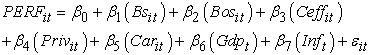(1)

Where PERFit is the performance measure of the ith individual at time t. This dependent variable will be measured by: ROA, ROE, and PB, β0 is the intercept (the constant of the model) and βk, k=1,...,7 are the coefficients to be estimated, and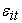is the error term. A significantly positive coefficient estimate signifies a statistically significant positive relationship between the bank profitability and the corresponding determinant. In contrast, a significantly negative coefficient estimate signifies a statistically significant negative relationship between the bank performance and the corresponding determinant.

Indeed, since three performance proxies are taken, we will have three models to estimate. Each model will be estimated using static panel-data estimation method. For each model regression, the random effect (RE) vs the fixed effect (FE) will also be tested via the Hausman test{6}.

5.1. Model 1

The first model will study the statistical relationship between the dependent variable ROA and the seven candidate exogenous variables. Its equation is as follows: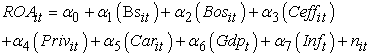(2)
5.2. Model 2

The second model will test the statistical relationship between the dependent variable ROE and the seven candidate independent variables. Its equation is as follows: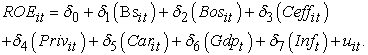(3)
5.3. Model 3

The third model will assess the statistical relationship between the endogenous variable PB and the seven candidate explanatory variables. Its equation is as follows: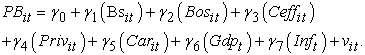(4)

6. Empirical Results and Findings

For the first model as described by equation (2), we run the Hausman test. As shown, in the Table 5 below, the p-value is equal to 0.1666. It follows that we do not reject the null hypothesis that there is no misspecification. Consequently, the model 1 will be estimated using the RE.

As shown, in Table 6 below, the estimation of the underlying model 1 leads to the following results.

According to the model estimation output above, all the explanatory variables, in model 1, are statistically insignificant given that all p-values are greater than 10%. The intercept (the constant) is also statistically not significant. The model 1 is globally and statistically insignificant. Therefore, the ROA as a proxy of the bank performance is not statistically explained by the underlying candidate determinants. However, some coefficient estimate signs are in line with the corresponding hypotheses. It follows that some hypotheses are partially accepted. In fact, Bos, Ceff, Car, and Priv are not statistically significant, but their coefficient estimate signs are as predicted by the hypotheses, respectively, H1, H3, H4, and H5. We recapitulate, in the Table 7 below, the rejected and partially accepted hypotheses.

For the second model as described by equation (3), we run the Hausman test. As shown, in the Table 8 below, the p-value is equal to 0.6042. It follows that we do not reject the null hypothesis that there is no misspecification. Consequently, the model 2 will be estimated using the RE.

As shown, in Table 9 below, the estimation of the underlying model 2 leads to the following results.

According to the model estimation output above, the intercept (the constatnt), Bs, Bos, and Inf are statistically significant, respectively, at 1%, 5%, 1%, and 10% level. However, only the Bos coefficient estimate has a sign in line with its corresponding hypothesis. The model 2 is globally and statistically significant. As a result, the ROE as a bank profitability measure is statistically explained by Bs, Bos, and Inf. We recapitulate, in the Table 10 below, the accepted, partially accepted, and rejected hypotheses.

For the last model as described by equation (4), we run the Hausman test. As shown, in the Table 11 below, the p-value is equal to 0.6270. It follows that we do not reject the null hypothesis that there is no misspecification. Consequently, the model 3 will be estimated using the RE.

As shown, in Table 12 below, the estimation of the underlying model 3 leads to the following results.

According to the model estimation output above, the intercept (the constant), Bos, and Priv are statistically significant, respectively, at 1%, 1%, and 10% level. In addition the Bos and Priv signs are as expected to be. the model 3 is globally and statistically significant. Consequently, the PB as a bank performance proxy is significantly explained by Bos and Priv. We recapitulate, in the Table 13 below, the accepted, partially accepted, and rejected hypotheses.

Amongst the estimated three models, we eliminate the first one because it is statistically and globally insignificant. The remaining models are models 2 and 3 wherein, respectively, the bank performance is measured by ROA and PB. Our selection criterion is the adjusted-R². Model 2 has an adjusted-R² equal to 0.054705, while model 3 has an adjusted-R² equal to 0.095595. As a matter of fact, we choose the model 3 because it has the greatest adjusted-R².

Given that our sample consists of private and state-owned banks as well and it covers a period from 1997 to 2015 where 2011 (the year of revolution) is inside the sample period, we suspect that the presence of state-owned banks in our sample disturbs our empirical results (sub-hypothesis Ha), and so does the year 2011 (sub-hypothesis Hb). Thus, we re-estimate the models excluding the state-owned banks from our initial sample, and we run the same models for the sub-periods: before 2011 and after 2011.

Starting with the second model, the explanatory variable Bos becomes statistically insignificant when we remove the state-owned banks from our sample. It also becomes reliably insignificant following the cut-off point year 2011. Consequently, both hypotheses Ha and Hb are empirically confirmed. Moving to the third model, the independent variable Bs becomes statistically significant after the breaking point 2011, which confirms H3.

All in all, after testing our two sub-hypotheses Ha and Hb, we point out the following empirical results: (a) state-owned banks included in the sample disturb the statistical significance of the results and (b) the year 2011 is a cut-off point that changed the Tunisian bank performance determinants.

7. Conclusion

Based on our empirical findings, the explanatory variable Bos is found out to be statistically and negatively correlated to the bank performance measured by ROE and PB within panel-data model regressions using random effects (RE). In fact, the more number of officials are present in the board of directors, the lower is the bank performance and vice versa. Consequently, Tunisian quoted banks should reconsider their governance structure. From the descriptive statistics, we report that the average number of directors is equal to almost 11. Instead of leading to wiser decision making, a larger board would result in ineffective initiatives. Based on our analysis, Following the cut-off point 2011, the determinants of Tunisian listed banks changed in terms of statistical significance and coefficient sign. Therefore, it is fundamental for these financial institutions to take into account this fact whenever conducting a performance analysis that covers a period prior or including the breaking point year 2011. In case of performance determinants considered individually has not changed, it is important to update them using data starting from the year 2012. Starting from 2012, the explanatory variable Bs becomes statistically significant and is positively correlated to bank performance. It means that the higher is the bank size the better is the bank performance. Therefore, directors should engage in better procedures in order to raise the value of their total assets. This further confirms the literature of Spathis et al 59 who investigated the effect of large bank size on Greek bank profitability from 1990 to 1999. They found that both had a positive relationship. Finally, the exogenous variable Priv is evidenced to be statistically significant and positively correlated to bank performance. Therefore, State-owned banks should reconsider the option of being privatized, especially that we evidenced their disturbance effect on our studied sample.

Acknowledgements

We would like to thank an anonymous referee for helpful comments and criticism. We also owe a great deal of gratitude to those who have helped us along this research.

Notes

1. Especially in the context of Tunisia, the environment is turbulent and marked by political unrest.

2. Net interest margin is a performance ratio that evaluates the goodness of investment considering the debt amount of a firm. It is equal to the difference between investment return and Interest expenses divided by the average earnings assets.

3. Middle East North Africa.

4. The market price used to calculate the price to book ratio is the close price on December 31st.

5. The book value per share (BVPS) is calculated as follows: (Total assets-Total liabilities)/Total number of shares outstanding.

6. We use EViews to run the underlying regresion equation estimations and related tests.

ReferencesThis work is licensed under a Creative Commons Attribution 4.0 International License. To view a copy of this license, visit http://creativecommons.org/licenses/by/4.0/